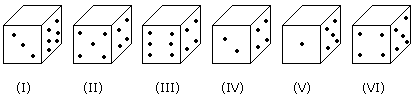# Verbal Reasoning - Dice - Discussion

### Discussion :: Dice - Dice 2 (Q.No.5)

Six dice with upper faces erased are as shows.The sum of the numbers of dots on the opposite face is 7.

5.

If the dice (I), (II) and (III) have even number of dots on their bottom faces, then what would be the total number of dots on their top faces?

 [A]. 7 [B]. 11 [C]. 12 [D]. 14

Explanation:

No. of dots on the top faces of dice (I), (II) and (III) are 5, 1 and 5 respectively.

Required total = 5 + 1 + 5 = 11

 Ritu Raj said: (Oct 11, 2015) Can you please elaborate the method to find its answer?

 Shannu said: (May 18, 2016) Please explain the solution.

 Ravali said: (Jun 17, 2018) Here they said the sum of each opposite side should be 7. So, for the first cube, it is given 3 and 6 dots so we have to check by combing with which number it will give 7 that will be the opposite of the number so, For Ist cube, it will be (3,4),(6,1)(2,5), IInd cube (5,2),(4,3),(6,1), IIIrd cube (6,1),(4,3),(2,5), IVrth (2,5),(4,3),(6,1), Vth (1,6),(5,2),(3,4), VIth (4,3),(5,2),(6,1), Starting from the first problem take this coordinates and u get the answers. for the 1st problem they asked even number cube has even num on top. So 2,4,6 are even cubes only the third coordinates can be on top and bottom. In the Q1 even should be present so it is 6.in the same way we get 6 for both 4 and 6th cube and adding those 6+6+6=18. Now this problem they gave !, II, III have even num of dots i.e.,2,6,2. then the bottom will be 5,1,5 so adding this 5+5+1=11 which is the answer .